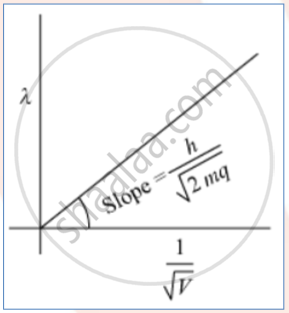# Plot a Graph Showing the Variation of De Broglie Wavelength (X) Associated with a Charged a Particle of Mass M, Versus 1 √ V Where V - Physics

Diagram
Short Note

Plot a graph showing the variation of de Broglie wavelength (X) associated with a charged a particle of mass m, versus  1/sqrtV where V is the potential difference through which the particle is accelerated. How does this graph give us information regarding the magnitude of the charge of the particle?

#### SolutionThe slope of the graph is h/sqrt(2mq)considering we know the slope and mass of the charged particle we can easily calculate the charge.

Concept: De Broglie’S Explanation of Bohr’S Second Postulate of Quantisation
Is there an error in this question or solution?